# Collision of the essentials of the conical sections // Hyperbola(Edited)

Greetings partners of the community of steemit, welcome again to my blog, where he can find content to the wide universe of the science, mathematics, biology, physics, chemistry, philosophy and the general accompanied knowledge of the scientific publication, specially greetings to the community of #steemstem.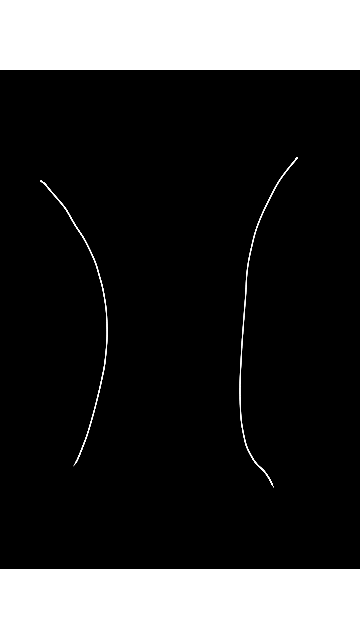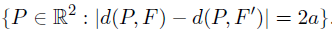in previous opportunities to share the content of the sections of the conical one, mentioned: to the circle, ellipse, parable and in this opportunity I will share them the content of the hyperbola and his importance, additional his applications since important in the field in the science and in other disciplines.

Now partners it is interesting to us to know the definition of the hyperbola at level of the sections of the conical one, where this one can be F1 and F2, what goes to represent two points in the plane, where to representa to a constant of positive value, which the hyperbola also they can be the set of point so much for (x, y), which is represented in the plane, since the difference of his distance pair F1 with F2 goes to be 2a. Where we have the following thing left clear: 2nd

Remember that the conical one determined by means of the conditions / PF / =e / PL / is an ellipse if 0 <and <1="" and="" a="" hyperbola="" if="" e=""> 1, in any case, the conical one has two apexes, which we call A ´ and A, the point of the axis biggest than the half of the distance between A ´ and A is the center of the conical one. The ellipse and the hyperbola are symmetrical with regard to his center it is called conical head offices.
Information consulted in Calculation Edwin Joseph Purcell, ‎Steven E. Rigdon, ‎Dale E. Varberg, 2007.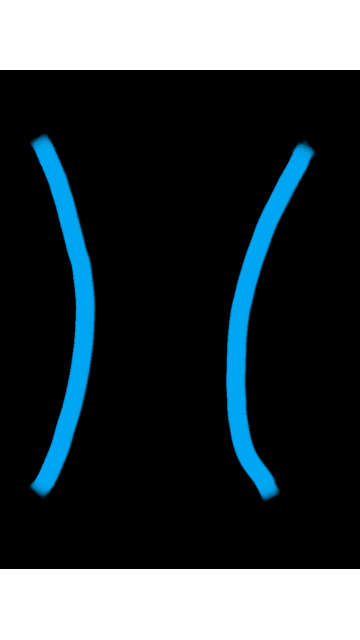I want to share following partners lover dela scientific publication, realizing already well the definition dela hyperbola we can tackle the following example, like part of his application as it it is the focal property of it served as base for the construction of telescopes, taking a common telescope as a reference guy Cassegrain, in a simple way this one consists of a primary parabolic mirror of form and of a secondary hyperbolic mirror of form, the conical combination of sections is a tool in the field of the astronomy additional to these the reflective property of the hyperbolas is used also in telescopic lenses.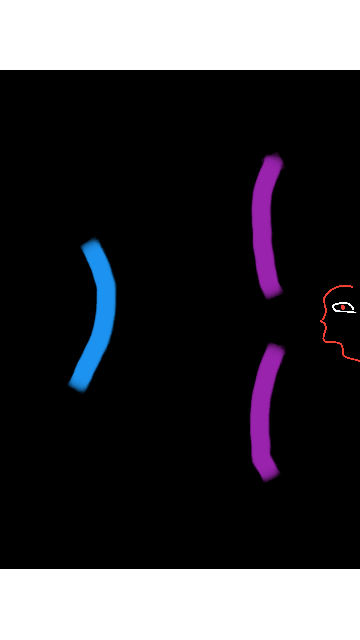primary mirror(parabolic).

 secondary mirror(hyperbolic). A representation Telescope Cassegrain.

he will wonder about that this representation talks each other, good I explain to them de the following way, the light this one is reflected so simply in a primary parabolic mirror and then this it is projected in other in his focal axis (F), but to the moment to come the light comes to a lens of form of hyperbola, making possible a symmetry of two focus of two sections conical of a parable and a hyperbola, where there is the observed one, which by means of the reflection of the light of the glasses, distances can receive the images located in big one.

Ax^2+Bxy+Cy^2+Dx+Ey+F=0. In the Cartesian plane of any equation in which A, B and C they they all are not zero and we demonstrate that almost always it is a conical section, the exceptions are between others the cases in which the graph does not exist or this one consists of two straight line parallel. By convention all the graphs of the equation, curled or not, are named a curve cuadráticas.
Information consulted in Calculation: several variables for George Brinton Thomas, 2006.

Every hyperbola has an eccentricity, hyperbolas it is seen that a few it forms where it is demonstrated more open than different, this detail is a part, since characteristic measures itself to a number called eccentricity (e), that is the quotient of c between to: and = c / to, with c> to, who must know each other like c> to, deduces that the eccentricity of the hyperbola is a number <1 at level of mathematical equation or mathematical model to give an explanation that depends his forms more open than different where and = c/a, (c) represents the half of the distance of the focal axis, (a) represents the half of the distance of the biggest axis.

I end with following reading friend for the case of the hyperbolas they have a practical use in the field of the optics and the astronomy, which they serve to study the massive bodies that interact thank you the law of universal gravitation, in which it describes in an easier way his trajectories in which they describe the conical sections, in which his center of mass is identified which this is at rest. at level of the geometric one.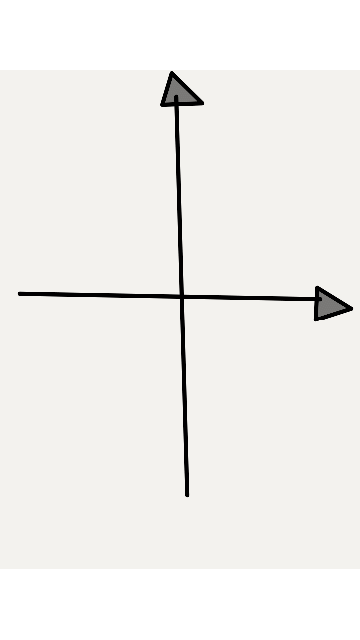(x-h)^2/a^2 – (y-k)^2/b^2 ordinary equation of the hyperbola which center is out of the origin. For the straight side of the curve in the hyperbola the expression is used: LR=2b^2/a, the relation between the quantities of to, b, y c they represent following values: (a) represents the distance of the center of the hyperbola to one of the apexes, (b) represents the distance of the center to an any end of the brought together axis, (c) represents the distance of the center to one of the foci.
Information consulted in analytical Geometry by Eduardo Carpinteyro Vigil, 2016.

All the images gif were prepared for @newton666, using the application Paperdraw.-Analytical Geometry by Eduardo Carpinteyro Vigil, 2016.

-Calculation: several variables for George Brinton Thomas, 2006.

- Calculation Edwin Joseph Purcell, ‎Steven E. Rigdon, ‎Dale E. Varberg, 2007.0
0
0.000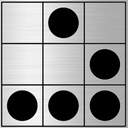This post has been rewarded with an upvote from city trail as part of Neoxian City Curation program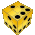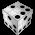. We are glad to see you using #neoxian tag in your posts. If you still not in our discord, you can join our Discord Server for more goodies and giveaways.

Do you know that you can earn NEOXAG tokens as passive income by delegating to @neoxiancityvb. Here are some handy links for delegations: 100SP, 250SP, 500SP, 1000SP. Read more about the bot in this post. Note: The liquid neoxag reward of this comment will be burned and stake will be used for curation.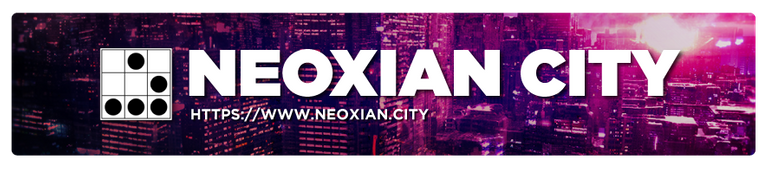0
0
0.0000
0
0.000@tipu curate

0
0
0.000Thank you for your support @eltonwright

0
0
0.000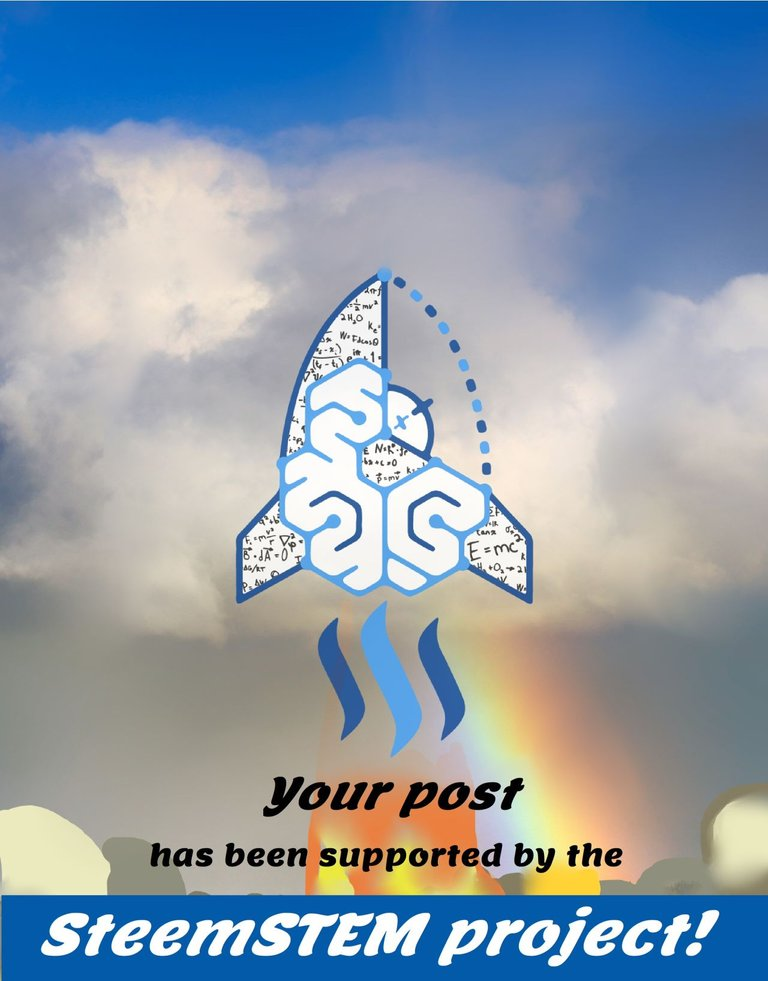This post has been voted on by the SteemSTEM curation team and voting trail. It is elligible for support from @curie and @minnowbooster.

If you appreciate the work we are doing, then consider supporting our witness @stem.witness. Additional witness support to the curie witness would be appreciated as well.